# 2004 Toyota Prius electric motor simulation using MotorAnalysis-PM

MotorAnalysis - Electric Machine Design and Analysis Software

This example presents the analysis of the 2004 Toyota Prius traction electrical motor using MotorAnalysis-PM. The simulation results are validated using measured data publically available in a series of reports published by Oak Ridge National Laboratory [1-3].

The 2004 Toyota Prius has a 8-pole permanent magnet motor with interior magnets. The single layer windings are made of 3 phases. The stator has 48 slots. The motor can deliver a maximum torque of 400 Nm and a peak-power output of 50 kW over the speed range of 1200–1540 rpm. The detailed design data of the motor can be found in the corresponding simulation file provided together with MotorAnalysis-PM.

Figure 1 shows the RMS line-to-line back-EMF vs. rotor speed obtained using the D-Q Analysis of MotorAnalysis-PM at zero stator current (open circuit). Since the stator current is zero, the winding and magnet temperatures for the simulation are assumed to be 20°C. The simulation results are showing the good agreement with the measured data from  shown in Figure 2.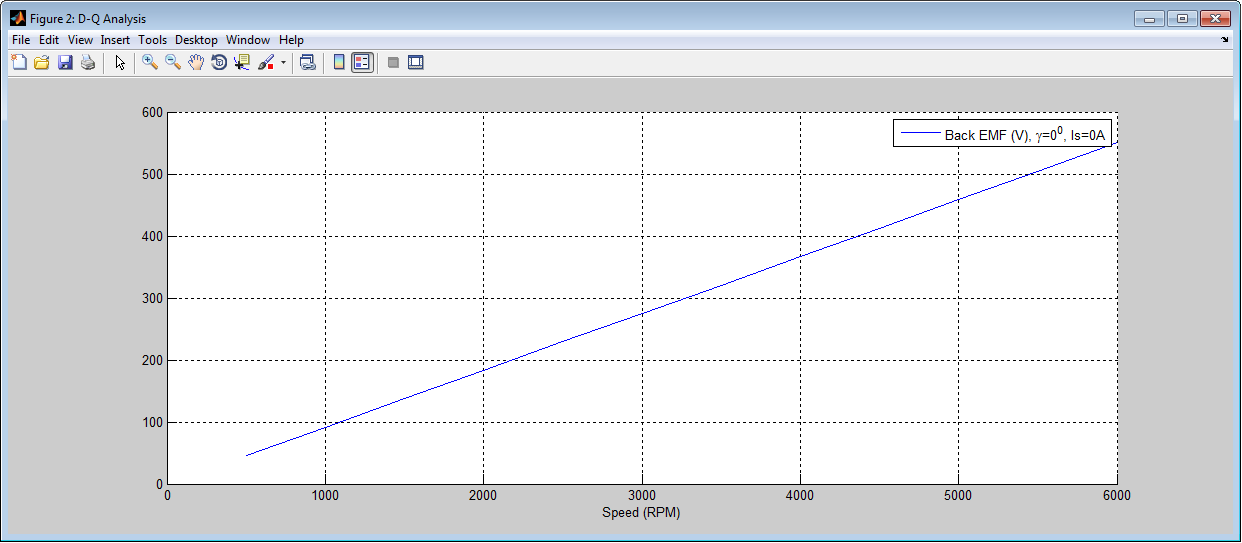Figure 1. Simulated RMS line-to line back-EMF vs. rotor speed.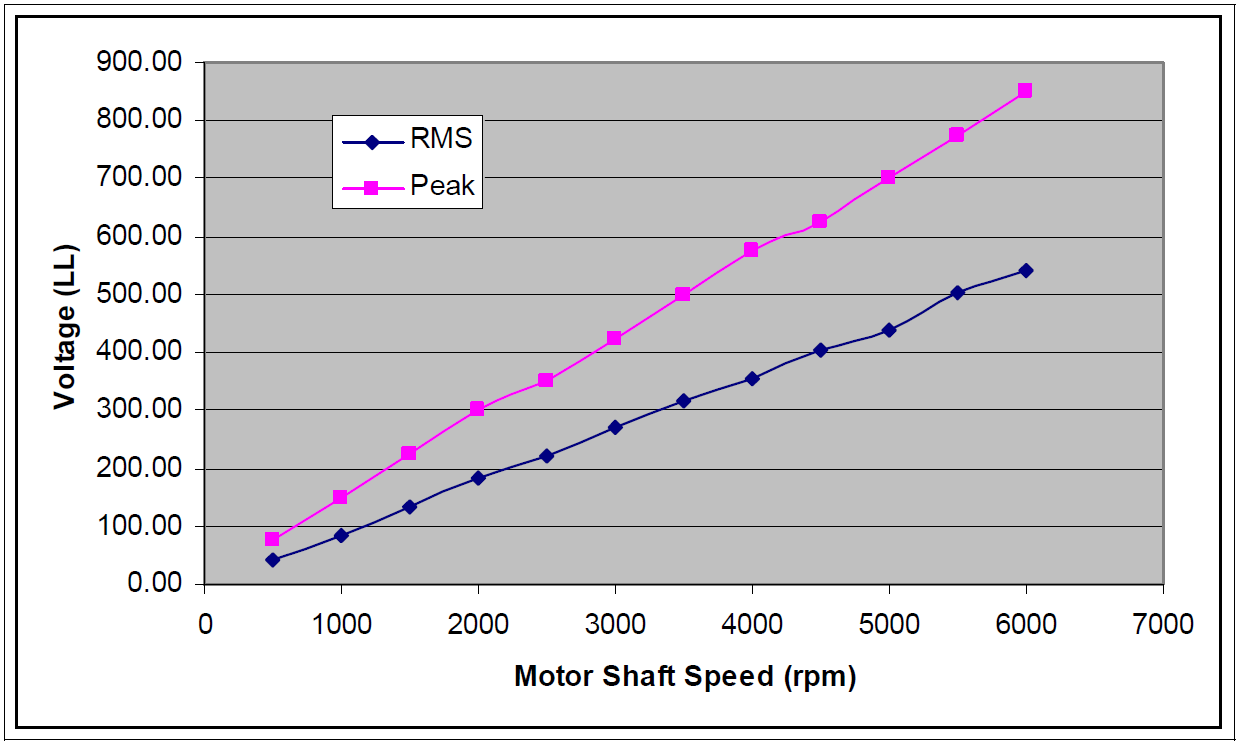Figure 2. Measured back-EMF vs. rotor speed.

The back-EMF waveform at 3000 rpm computed using a series of magnetostatic simulations for different rotor positions is shown in Figure 3. The measured back-EMF waveform at the same rotor speed found in  is presented in Figure 4.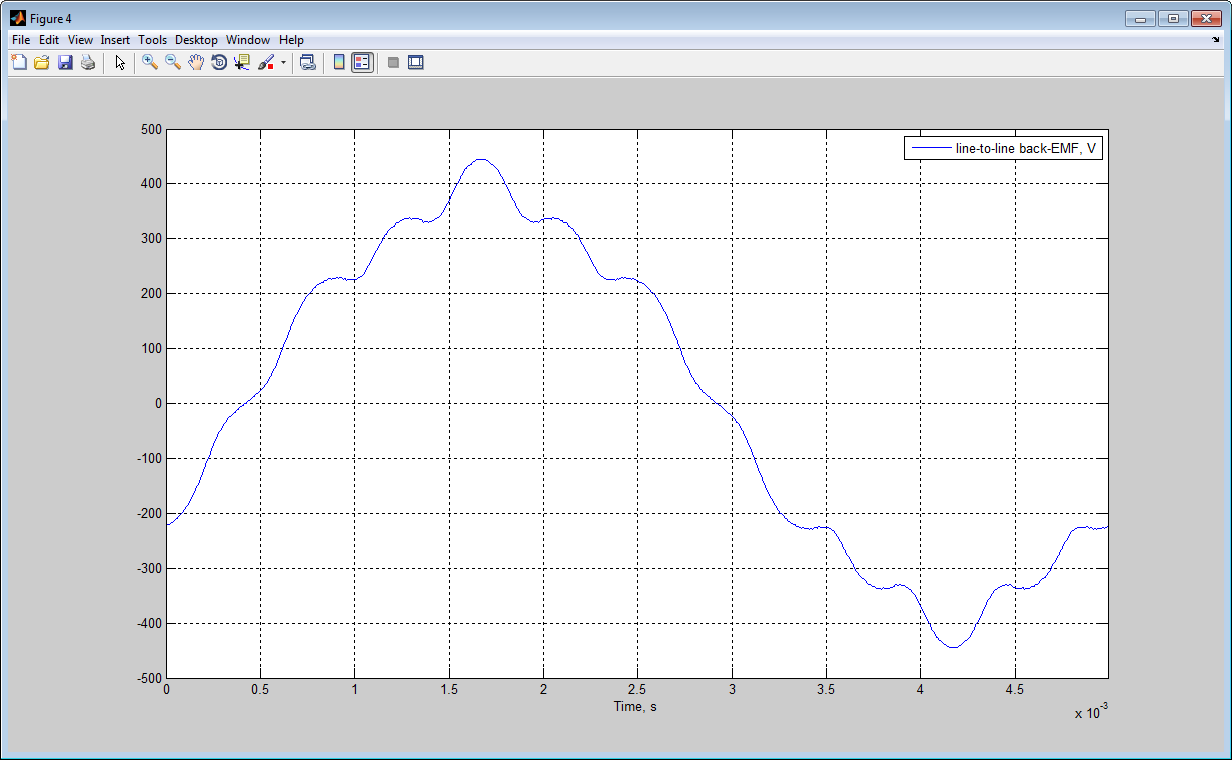Figure 3. Simulated line-to-line back-EMF waveform at 3000 rpm.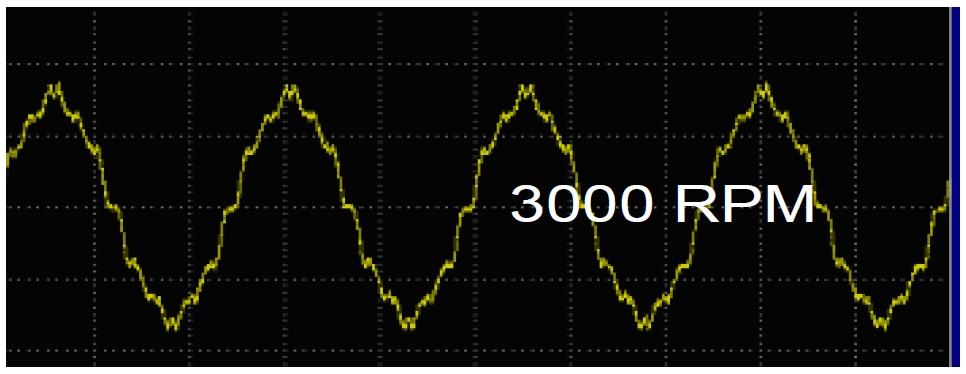Figure 4. Measured line-to-line back-EMF waveform at 3000 rpm.

To obtain the torque characteristics vs. advance angle the locked rotor test was performed and the measured results are presented in . Since the torque does not depend on speed, the D-Q Analysis of MotorAnalysis-PM was chosen to obtain the same torque characteristics from simulation results. Based on the thermal analysis data published in  the winding temperature of 160°C and the magnet temperature of 150°C were used for the simulations at load conditions. All simulation results presented below were obtained at these temperatures. The magnet remanence flux density was recalculated for 150°C using the remanence flux density temperature coefficient of the neodymium-iron-boron magnet -0.12 %/°C. Simulated torque vs. advance angle curves for different stator currents are shown in Figure 5 and the measured results of the locked rotor test from  are shown in Figure 6. Note that the DC current values used for the locked rotor test correspond to the peak AC current values used for the simulation. Not taking into account the distortions of the measured torque curves due to the slotting which are smoothed by the rotor rotation for simulated torque curves, the agreement between simulation and measured data is good. The slight discrepancy between simulated and measured curves at lower currents can be explained by lower magnet temperatures. To prove that, the additional torque curve at RMS current of 35.36 A (50 A peak) and magnet temperature of 20°C was computed. This torque curve shown in Figure 5 as a dotted line demonstrates the better agreement with the measured data.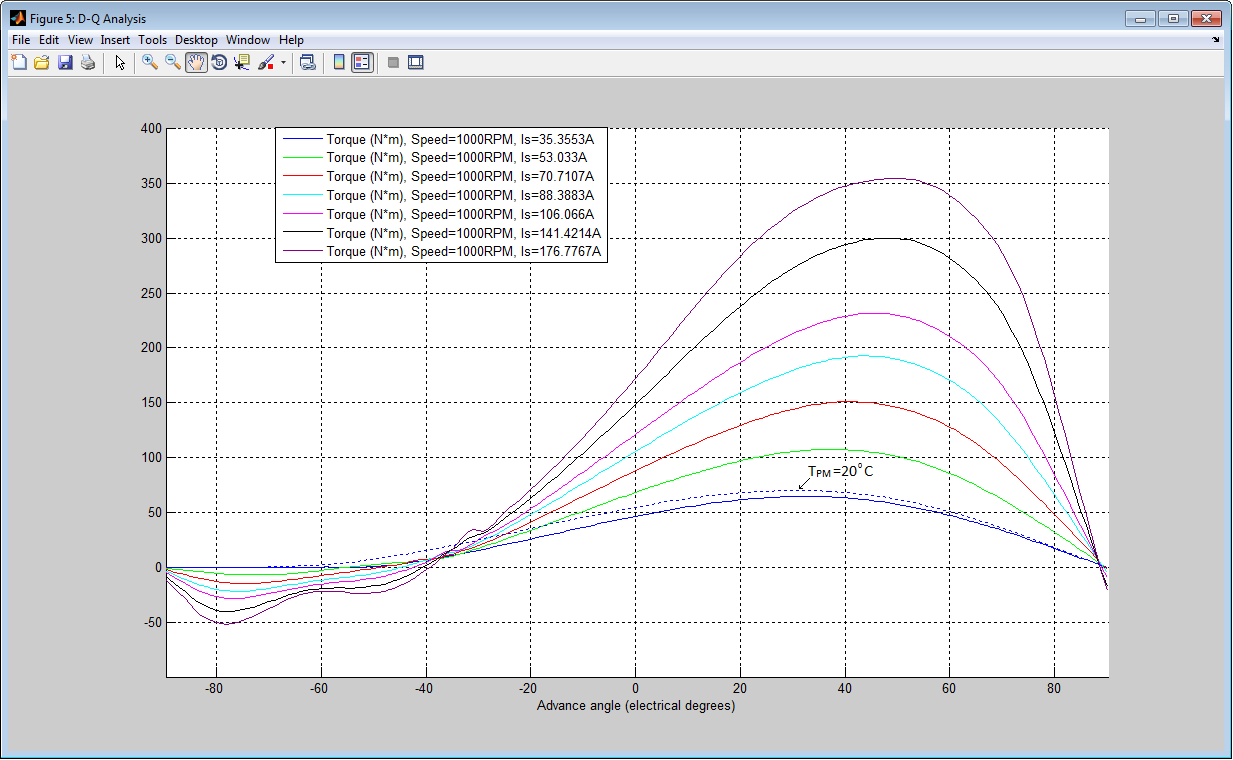Figure 5. Simulated torque vs. advance angle curves at various currents.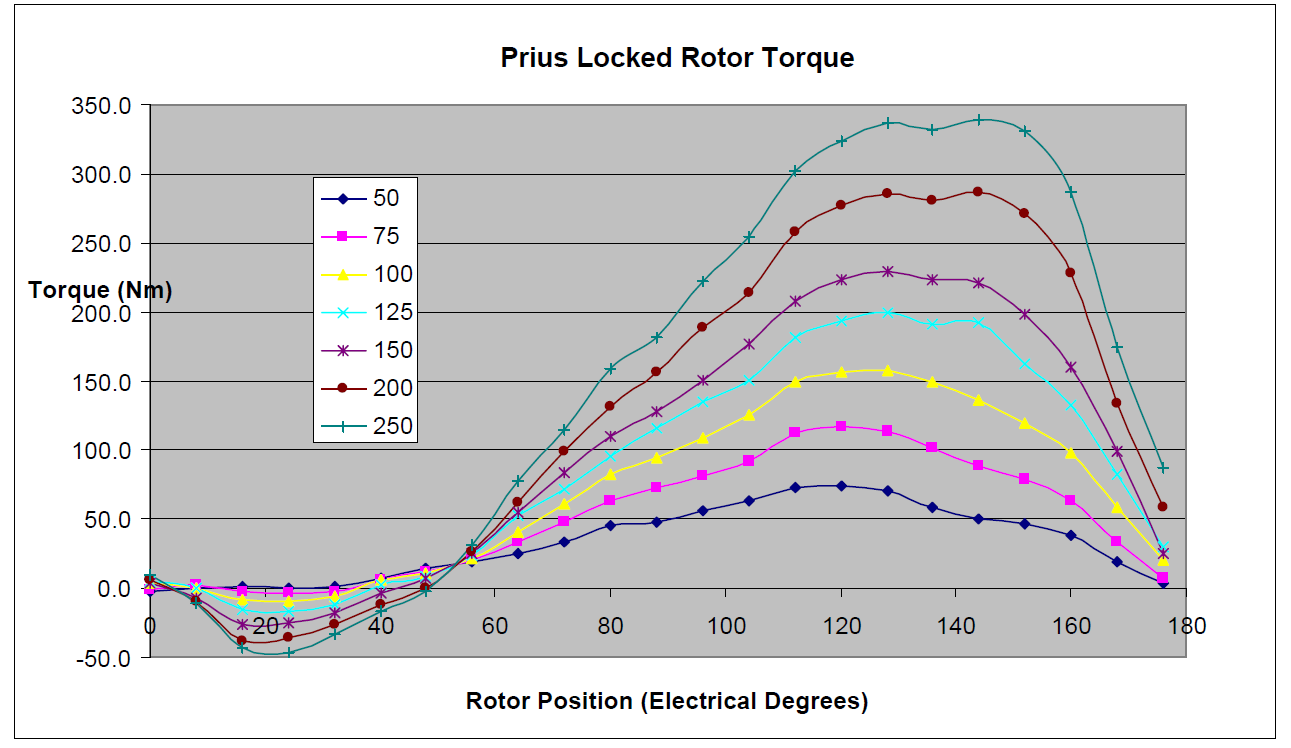Figure 6. Measured torque vs. electrical angle curves at various currents.

The further analysis determined that the current value of 216 A is required to generate the Prius motor maximum torque of 400Nm. As it can be seen from Figure 7 the advance angle for the maximum torque is 50 electrical degrees.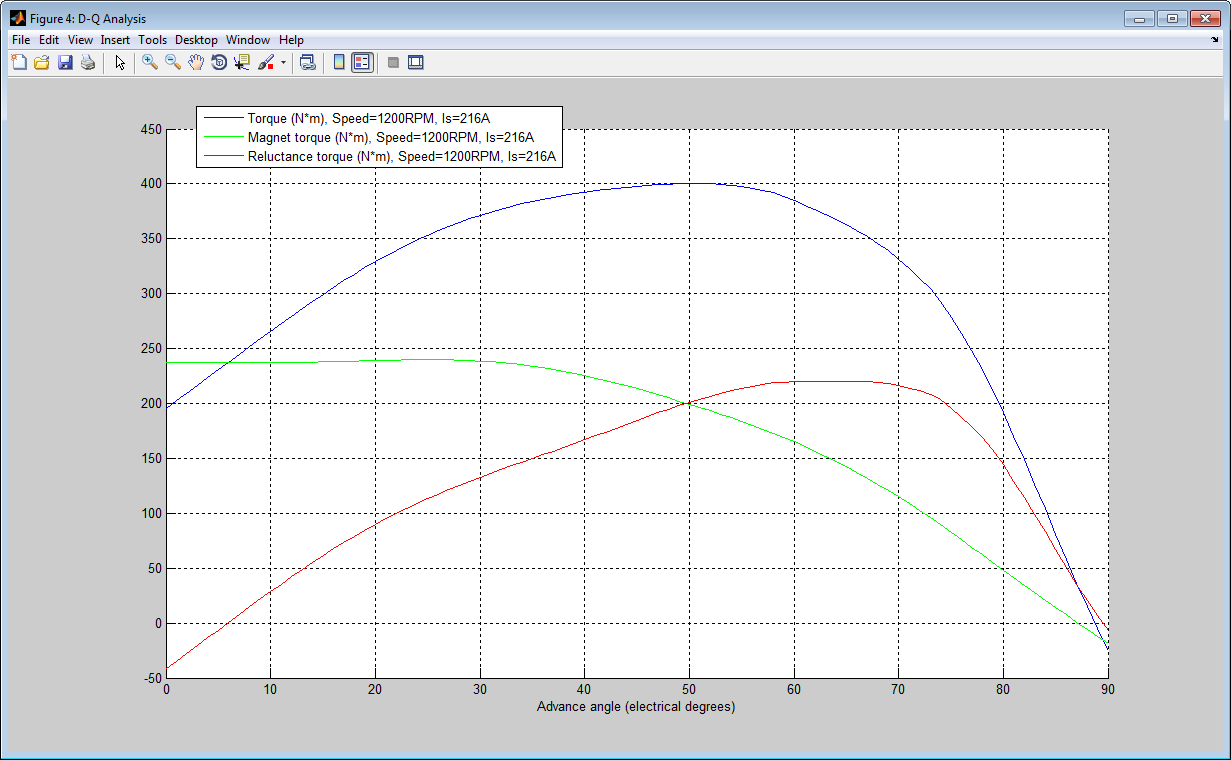Figure 7. Simulated torque curve with magnet and reluctance torque components vs. advance angle at 216 A.

Once the maximum torque current and advance angle are determined the torque-speed characteristic and some other important parameters of the Prius motor can be obtained using the D-Q Analysis of MotorAnalysis-PM. The resulting characteristics are shown in Figure 8. Field-weakening operation above 1200 rpm is determined to insure the maximum possible torque over the entire operating speed range of 0–6000 rpm. Maximum torque-speed performance specifications for the Prius motor found in  are shown in Figure 9.

The efficiency map of the Prius motor computed using the D-Q Analysis of MotorAnalysis-PM is shown in Figure 10. The mechanical loss data used for the efficiency calculation was taken from . The experimentally measured efficiency map published by ORNL in  is shown in Figure 11. As can be seen, the agreement between simulation and measured data is sufficient; both maps show efficiencies peaking at 93–94% in the 1500–3000 rpm range at torque levels 50–150 Nm.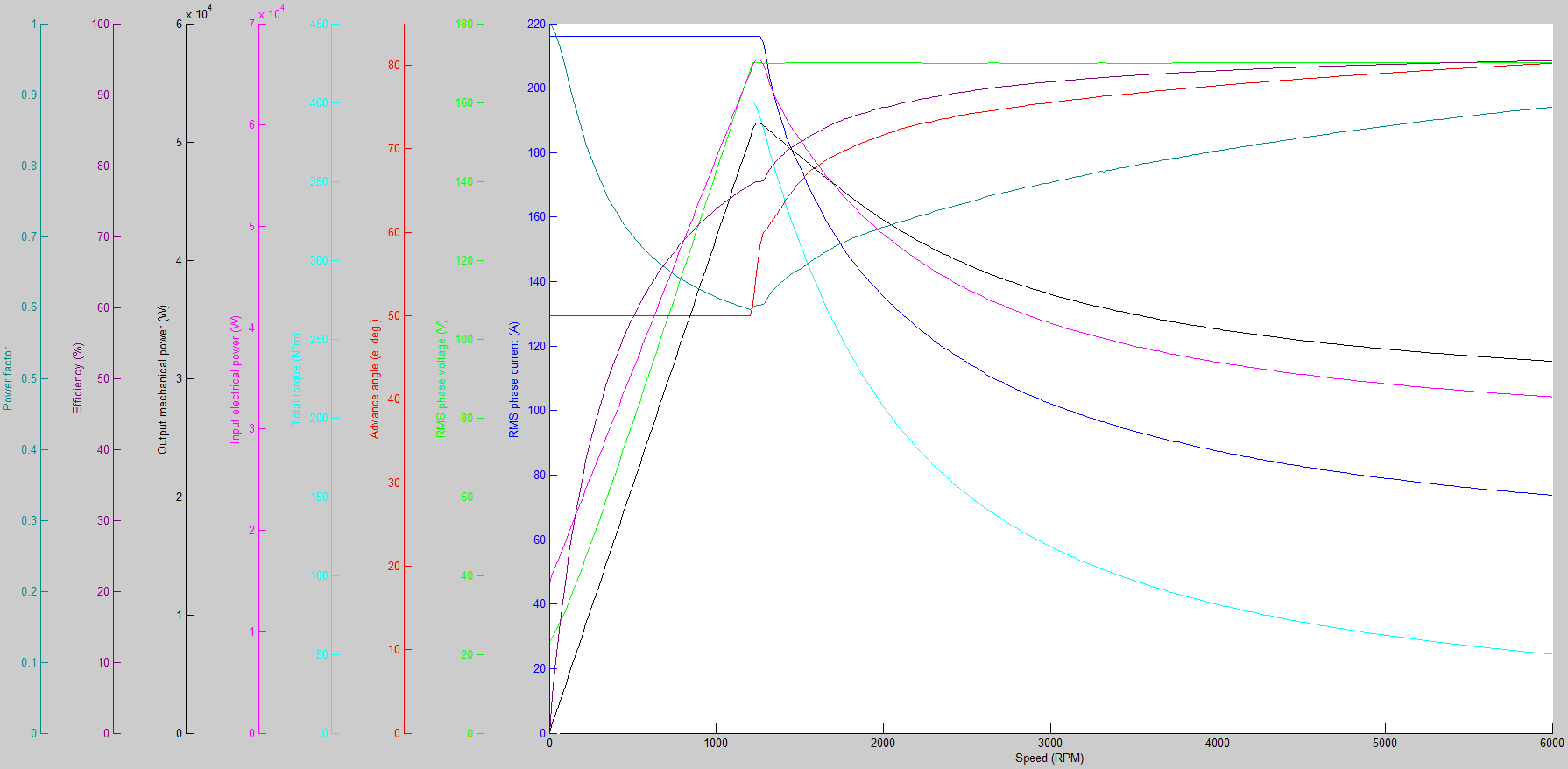Figure 8. Simulated torque-speed and other characteristics for the maximum torque operation.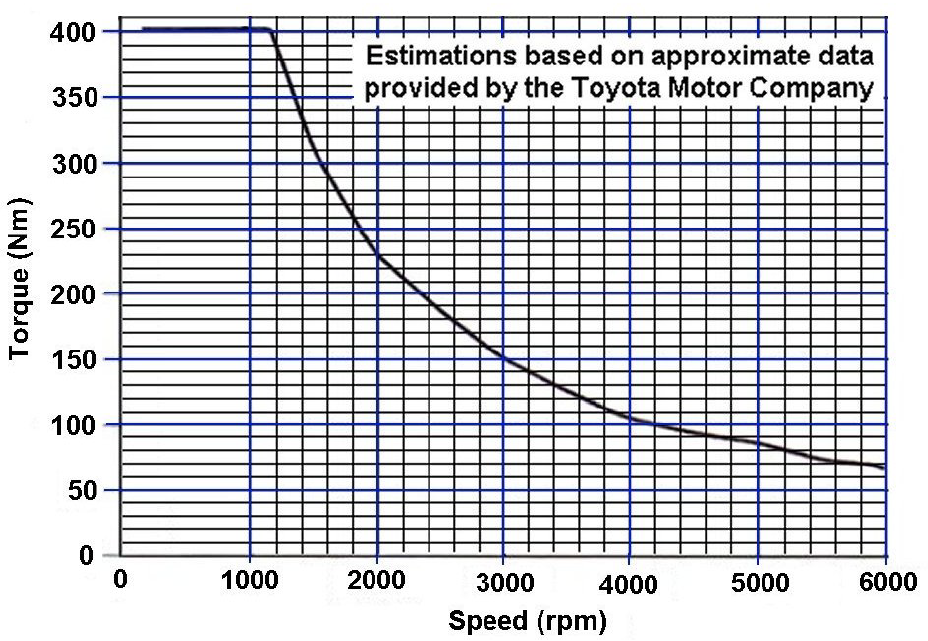Figure 9. Maximum torque-speed performance specifications for the Prius motor found in .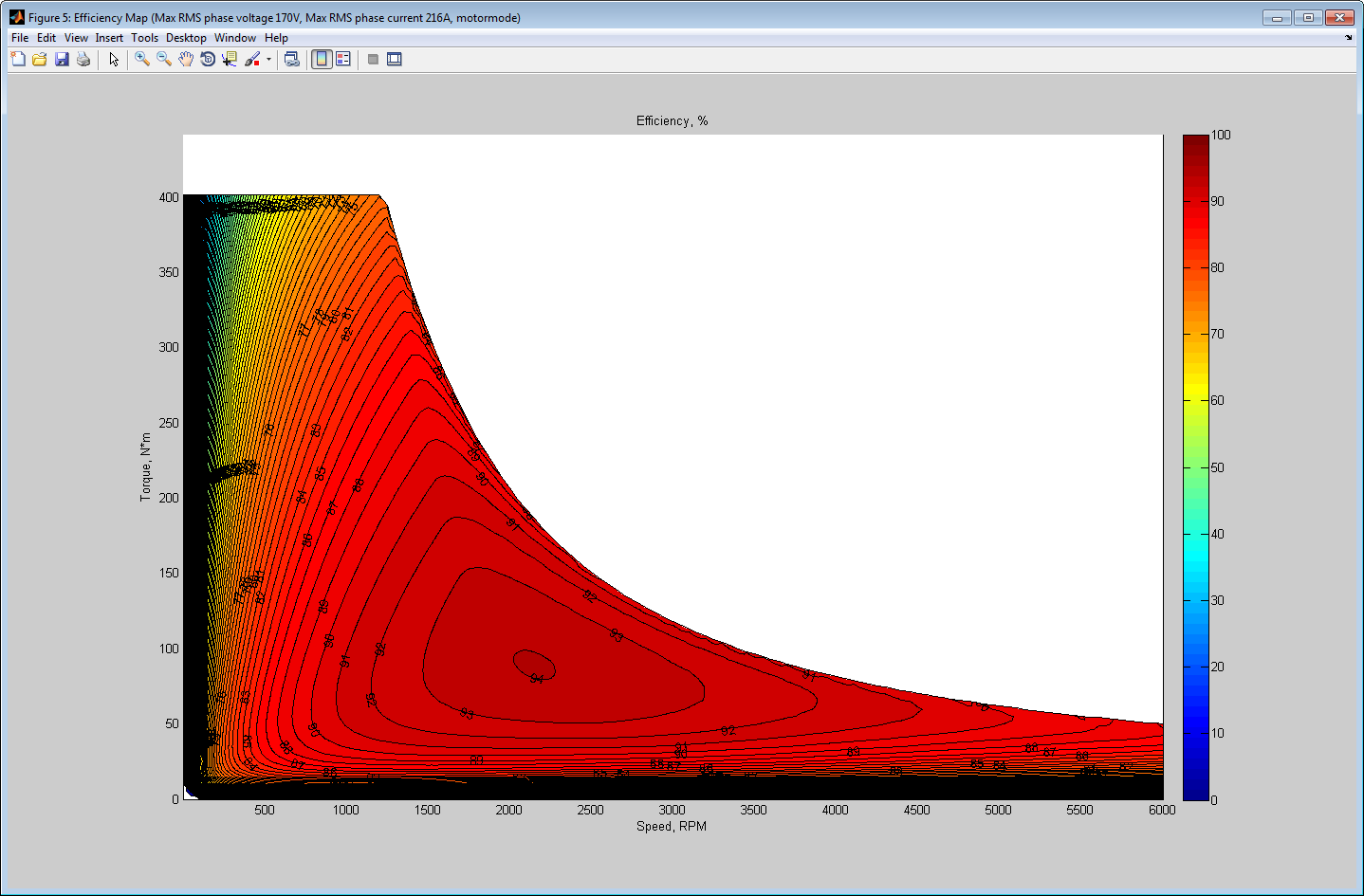Figure 10. Simulated efficiency map of the Prius motor.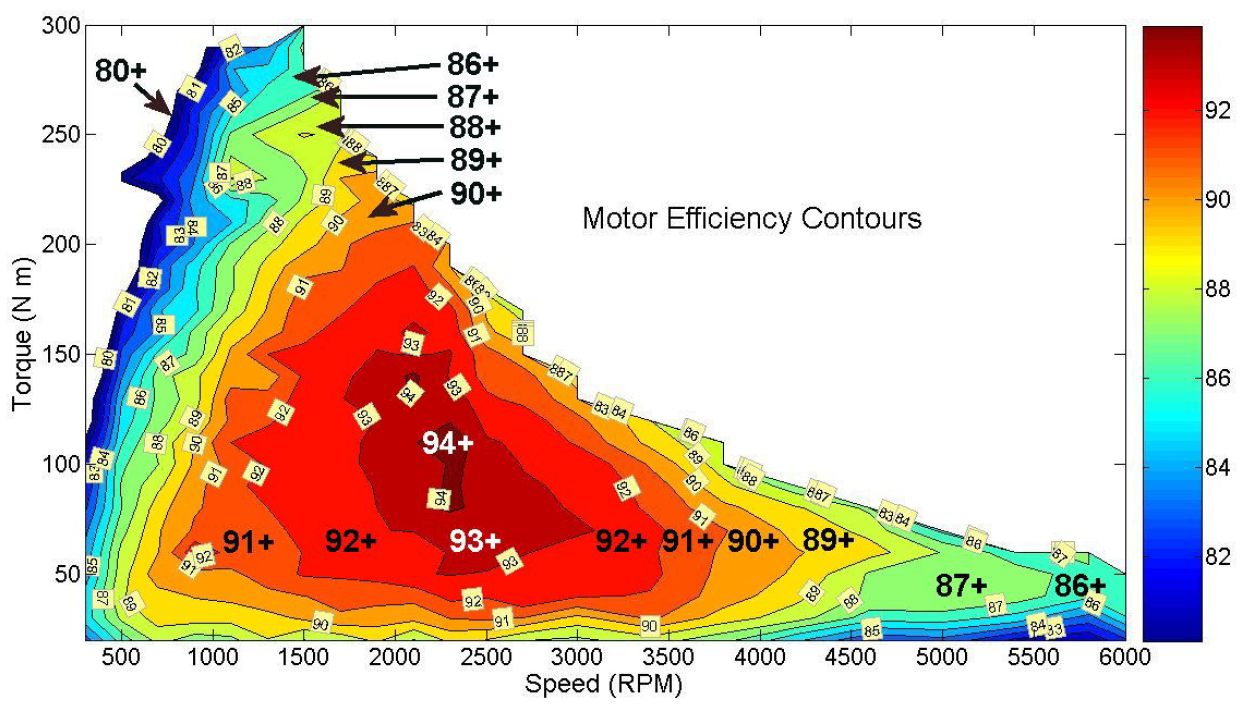Figure 11. Measured efficiency map of the Prius motor.

Finally, the dynamic finite element simulation of the motor driven from a three-phase voltage inverter at a fixed rotor speed of 1200 rpm was performed. The current hysteresis control was applied to provide the maximum torque operation at 216 A and advance angle of 50 electrical degrees. The resulting current, phase voltage and torque waveforms are shown in Figure 12, the time-averaged quantities are presented in Figure 13. The instantaneous flux density distribution of the Prius motor is shown in Figure 14.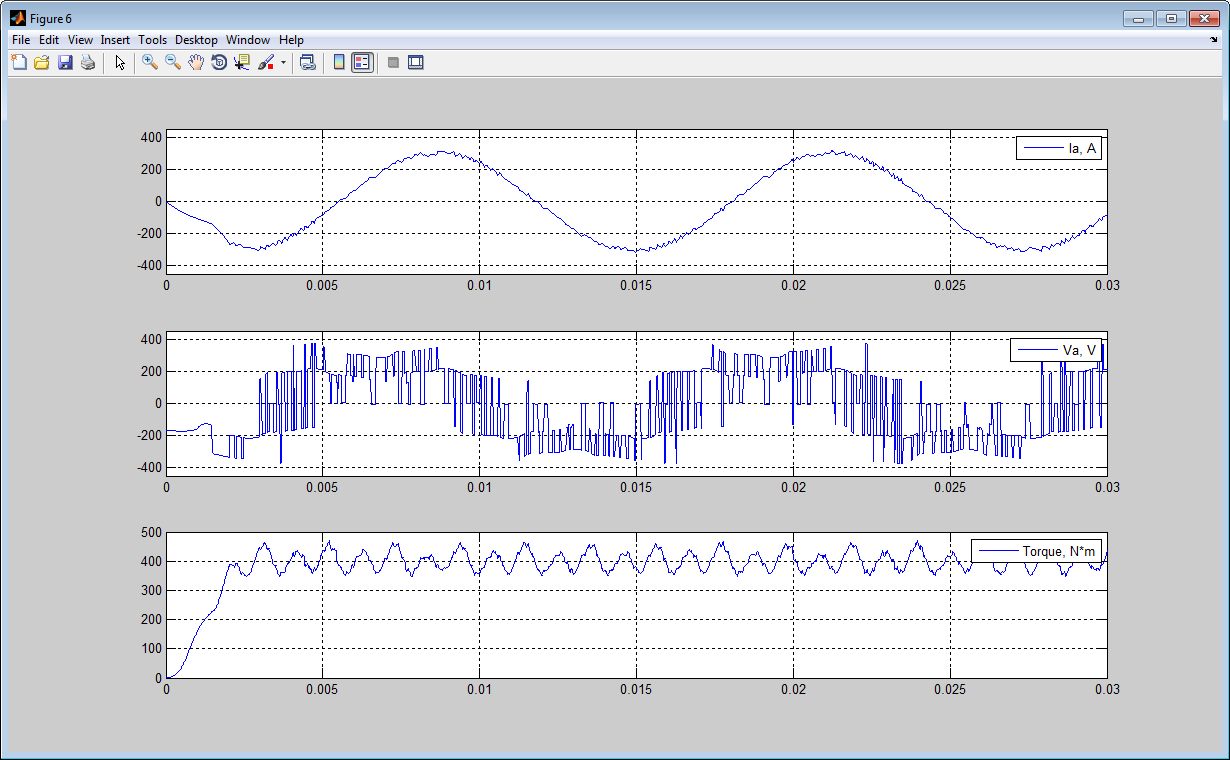Figure 12. Simulated current, phase voltage and torque waveforms at 1200 rpm.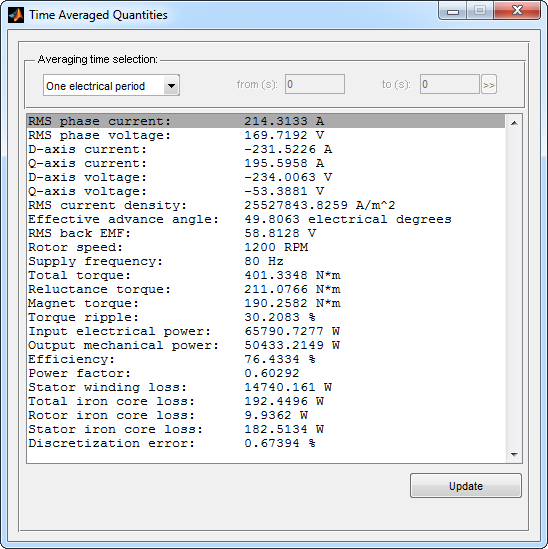Figure 13. Dynamic FE simulation time-averaged quantities.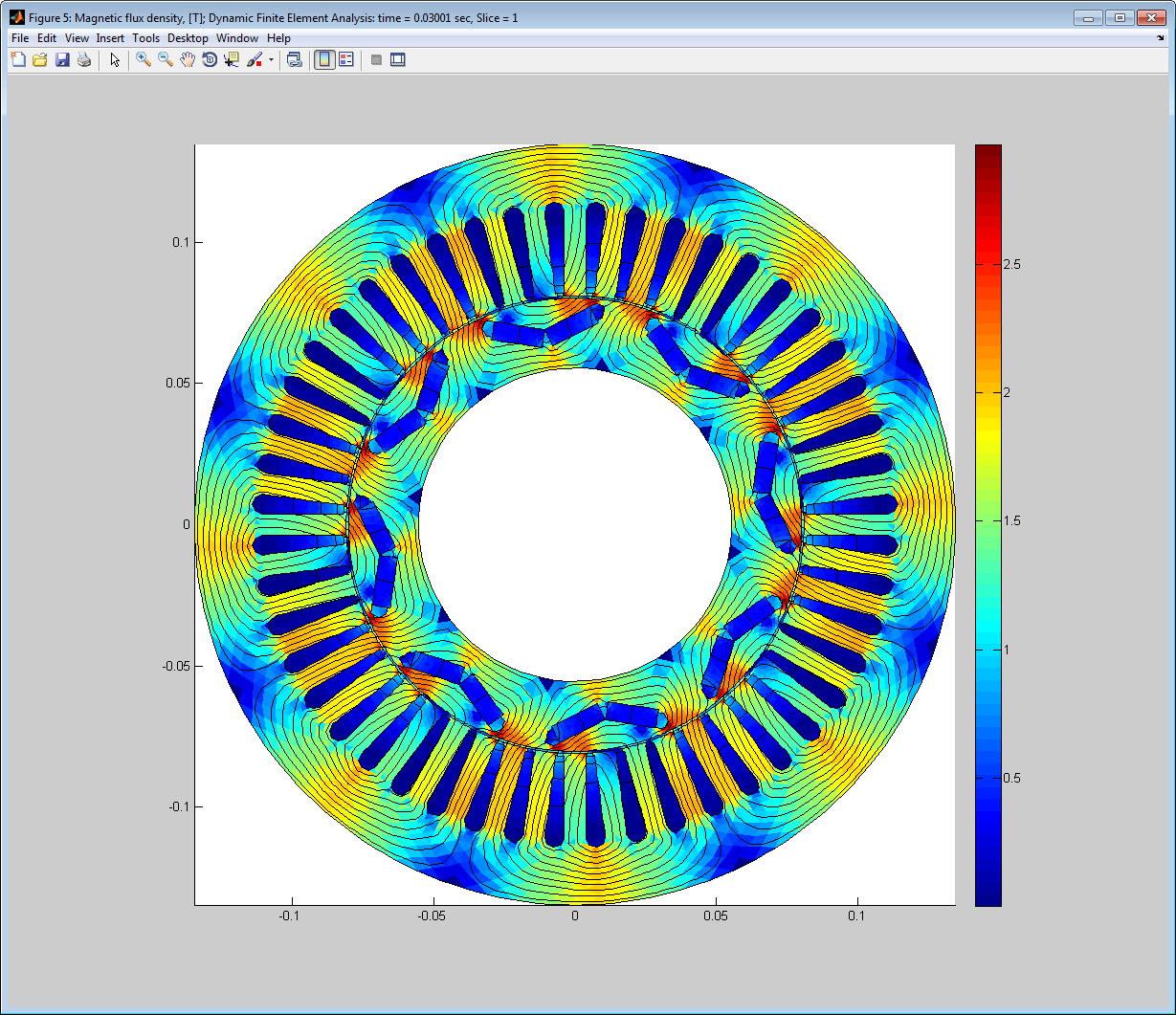Figure 14. Instantaneous flux density distribution of the Prius motor.

## References

 J.S. Hsu, C.W. Ayers, C.L. Coomer, R.H. Wiles, T. A. Burress – REPORT ON TOYOTA/PRIUS MOTOR TORQUE CAPABILITY, TORQUE PROPERTY, NO-LOAD BACK EMF, AND MECHANICAL LOSSES, Oak Ridge National Laboratory, ORNL/TM-2004/185
https://www.osti.gov/scitech/servlets/purl/921782

 J.S. Hsu, S.C. Nelson, P.A. Jallouk, C.W. Ayers, R.H. Wiles, S.L. Campbell, C.L. Coomer, K.T. Lowe, T.A. Burress – REPORT ON TOYOTA PRIUS MOTOR THERMAL MANAGEMENT, Oak Ridge National Laboratory, ORNL/TM-2005/33
http://info.ornl.gov/sites/publications/Files/Pub57487.pdf

 R.H. Staunton, C.W. Ayers, L. D. Marlino, J.N. Chiasson, T.A. Burress – EVALUATION OF 2004 TOYOTA PRIUS HYBRID ELECTRIC DRIVE SYSTEM, Oak Ridge National Laboratory, ORNL/TM-2006/423
https://www.osti.gov/scitech/servlets/purl/890029